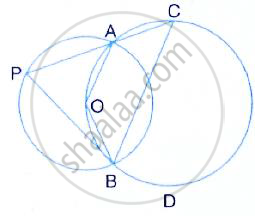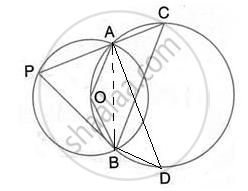Share

# The Figure Shows Two Circles Which Intersect at a and B. the Centre of the Smaller Circle is O and Lies on the Circumference of the Larger Circle. Given ∠Apb = A°. Calculate, in Terms of A°, the - Mathematics

Course

#### Question

The figure shows two circles which intersect at A and B. The centre of the smaller circle is O and lies on the circumference of the larger circle. Given that ∠APB = a°.

Calculate, in terms of a°, the value of : obtuse ∠AOB,#### SolutionObtuse ∠AOB = 2°∠APB = 2a°

(Angle at the centre is double the angle at the circumference subtended by the same chord)

Is there an error in this question or solution?